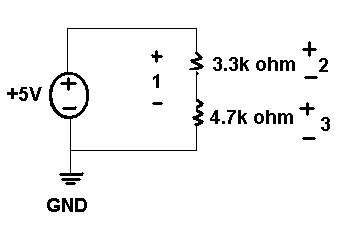# Digital Multimeters (DMMs) and Precision DC Sources

cancel
Showing results for
Did you mean:

## DMM current measurement problems

Hi.  I am a TA at the Unv of Florida and we are currently using the NI-ELVIS equipment.  I noticed one problem.  When trying to measure DC current using the DMM the value is not correct.  For example, a simple series circuit with a resistor of 3.3 k ohms and 4.7 k ohms and 5 V source will result in a current of about .625 mA however, depending upon where we break the circuit we get different measurements each time.  This happens on all of the stations in the lab (about 6).  Using a fluke the current measurement is fine.  Any idea on the problem???
Thanks.
Message 1 of 4
(8,003 Views)

## Re: DMM current measurement problems

Hello pinnacle1406,

1. When you say the measurements are incorrect, what do you mean? I have attached a circuit diagram below. What are the values when measured at point 1 (across both resistors), point 2 (across 3.3k ohm resistor) and point 3 (across 4.7k ohm resistor)?2. You said you tried the measurement on about 6 different NI ELVIS’s, are the measurements consistent on each station?

3. How are you measuring the current with the NI ELVIS? Are you using the Digital Multimeter Express VI in LabVIEW? Are you using the NI ELVIS Instrument Launcher?

4. What DAQ card are you using to take measurements from your NI ELVIS?

5. Are you using the proper null correction to lower the noise error?

From the NI ELVIS Suite data sheet, the current specifications are given as:

DC accuracy ........................................ 0.25% ±3 mA2

2Proper null correction at the common-mode voltage can reduce ±3 mA error to 200 μA noise.

Even with proper null correction, your current may be too small for the NI ELVIS to measure. If you are just trying to demonstrate the current dissipated in a simple circuit, I would recommend changing the resistor values so that you have a larger current running through your circuit.

Message Edited by Matt A on 01-18-2007 07:30 PM

Message Edited by Matt A on 01-18-2007 07:30 PM

Message Edited by Matt A on 01-18-2007 07:31 PM

Matt Anderson

Hardware Services Marketing Manager
National Instruments
Message 2 of 4
(7,989 Views)

## Re: DMM current measurement problems

Hello Matt_A

Depending on the figure you show, how will we measure current at different points?
I mean that to measure current at point 1, I put Current_HIgh at 1+ and Current Low at ground. Right?
For point 2, Current High at 2+ and Current low at Ground.

Now aren't the two cases same??

Secondly, I want to ask you, that suppose I have  a RC circuit and a known supply. The current in an RC circuit would vary. How can I measure the current in this case? I want to display the current measured on a chart from the time t=0 to the time the capacitor is fully charged. If I use the DMM vi present in the examples of ELVIS and plot the measured current on a strip chart, at what frequency will the strip chart plot the current i.e what is the rate of plotting? Can we control it? If yes, how? Can we attain the rate of 10 samples per millisecond in ELVIS I?

Message 3 of 4
(6,398 Views)

## Re: DMM current measurement problems

According to Kirchhoff's law, the current in a circuit is always the same, whereever you measure it, so of course you should get the same current reading whereever you measure it.

But... of course to insert a current measuring device you have to break the circuit at a certain point. So if you measure at 1, you probably break the circuit between the two resistors. But there is no place to break the circuit when doing the other measurements, or how and where do you break the circuit when measuring at location #2 or #3???

It would be helpful if you mark the places where you break the circuit to try to measure the current precisely (by an X across the wire).  Since a current measuring device has an impedance (input resistance) close to zero, you rather measure the short circuit current of your power supply when connecting it between location +2 and GND (you short both resistors), and you will get the current flowing through the 3.3kOhm resistor only when connecting it between location +3 and GND (since the 4.7kOhm resistor will be shorted by the current measuring device).

Message 4 of 4
(5,962 Views)